Search IntMath
Close

450+ Math Lessons written by Math Professors and Teachers

5 Million+ Students Helped Each Year

1200+ Articles Written by Math Educators and Enthusiasts

Simplifying and Teaching Math for Over 23 Years

# IntMath Newsletter: Log graphs, challenges, news

By Murray Bourne, 29 May 2019

29 May 2019

1. New on IntMath: Logarithm function from its graph
2. Breakthrough Junior Challenge
3. Resources: Wolfram, MathGraph32
4. Math in the news: Computers and equations
5. Math movies: Calculus, Probability
6. Math puzzle: Bowling
7. Final thought: extinctions

## 1. New on IntMath: Finding the logarithm function from its graph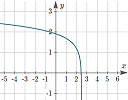This article explains how to observe the characteristics of a logarithmic graph to determine its equation. See: How to find the equation of a logarithm function from its graph?

## 2. Breakthrough Junior Challenge

One of the best ways to learn something is to explain it to someone else.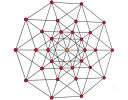The Breakthrough Junior Challenge encourages students to create a short explanatory video. Prizes include a \$250,000 post-secondary/college scholarship.

The overall idea, from the site:

Explain a big scientific idea in Physics, Life Sciences or Mathematics with a short video. You will have until June 15, 2019 at 11:59 PM PDT to submit your video (3:00 minutes max).

The number of submissions for math topics is quite low compared to the other categories. So, give it a go!

## 3. Resources

### (a) Wolfram Language WebinarsWolfram has released a new version of the Wolfram Language and Mathematica, and is offering 4 free Webinars to explain the new features.

I'm particularly looking forward to the June 12 one, which will cover:

Mathematics and Scientific Visualization
Calculus • Algebra • Complex Visualization • Geographic Visualization • Molecular Visualization

You can register here.

Of course, their main aim is commercial, but you can still learn a lot of worthwhile things by seeing how the experts make use of a powerful mathemtaical tool.

You may also be interested in Wolfram U, a series of videos which explores a range of math topics using Mathematica.

### (b) MathGraph 32 - updated to javascript

I've featured MathGraph32 a few times before on IntMath. It's a cross-platform, cross-browser interactive graphing solution, with some very nice features. It's similar in many ways to GeoGebra, but I find it easier to use for many cases.

From the developer, Yves Biton: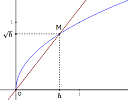"I have entirely rewritten MathGraph32 in JavaScript. It is still free under GNU GPL license. So no more use of Java, a new user interface without menu and new functionalities. Hope it will be useful because it was a tough job!"

MathGraph32 can be used:

1. Online (with easy to use menu icons); or

It also works on mobile devices.

Check out these tutorial videos in English, and here are some graph interactives.

## 4. Math in the news:

### (a) Using computers to crack open centuries-old mathematical puzzles

Throughout history, a lot of mathematical research ended up in the "too hard" basket, because it was just impossible to do all the calculations necessary to draw a conclusion.

One such example is that of Diophantine Equations, the most famous of which was xn + yn = zn, which has no whole number solutions when n is greater than 2, finally proved by Andrew Wiles after 300 years.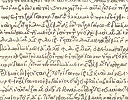Diophantus of Alexandria was a 3rd century BCE Greek mathematician, often called the "father of algebra", as a result of his books on algebraic equations, called Arithmetica, a snippet of which is shown here.

The article Using computers to crack open centuries-old mathematical puzzles by Christopher Rasmussen, Associate Professor of Mathematics at Wesleyan University, explains in layman's language what the researchers aimed to do by using computers.

The actual paper talks about how they used Sagemath, an open-source computer algebra system, to solve cubic Ramanujan-Nagell equations, for example:

x3 + 3k = qn, where q is an odd prime.

One such solution is x = 2, k = 4, q = 89 and n = 1, giving:

23 + 34 = 891

### (b) New memristor circuit solves linear equations in nanoseconds

An example of a simple linear equation written in matrix form is: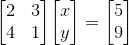(See Matrices and Linear Equations for background.)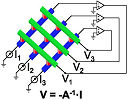A new circuit can solve large cases of such a system of linear equations in a single operation within a few tens of nanoseconds, much faster than using current or quantum methods.

It uses an analog method of in-memory computing, where one equation coefficient is stored as a unique resistance in one memristor (an electrical component that regulates the flow of current in a circuit and remembers the amount of charge that has previously flowed through it, retaining this memory even without power.)

The circuit was tested on a wide set of algebraic problems including:

1. Ranking of internet websites
2. Complex differential equations
3. Finding wave-function of an electron

## 5. Math Movies

### (a) Essence of Calculus - 3Blue1Brown

I've featured 3Blue1Brown's brilliant videos in earlier Newsletters. This time it's the series, Essence of Calculus.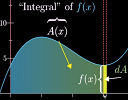If you are about to embark on studying calculus, this would be a great introduction:

### (b) False positive riddle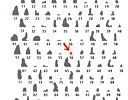Mining unobtainium is hard work – the rare mineral appears in only 1% of rocks in the mine. But your friend Tricky Joe has something up his sleeve (involving conditional probability).

## 6. Math puzzles

The puzzle in the last IntMath Newsletter asked about a general formula for a given sequence. There were an interesting range of responses.

Correct answers with sufficient reasons were submitted by Michael (who used modulus arithmetic) and Russell. Correct solutions (but with missing reasoning or details) were presented by: Gayathri, Christopher, Larry, Arun, and Nicola (who used a recursive approach).

### New math puzzle: Bowling

In his latest bowling game, Raj scored 199 and this raised his average over a number of games from 177 to 178. What must he score in the next game to raise his average to 179?

You can leave your response here. Hint: If you present your answer with full explanation of how you obtained it, then others can follow your thinking and learn something from it.

## 7. Final thought - extinctions

Some years ago I was on a retreat in Malaysia. I enjoy going for walks in the early morning, and one day I followed a track into the (secondary) jungle. I was quite surprised to come across recent evidence (just hours old) of elephants using the same track. It could have been dangerous had I met those elephants, but I was excited to know wild elephants were still in the area. (Most of the virgin jungle in that area has been cleared for palm oil production, so it was doubly surprising.)

So I was saddened by the death this week of the last captive male Sumatran rhino in Malaysia, the last in a country that used to have plenty of them.

There is a real insanity of animal destruction going on in order to feed the irrational ivory, rhino horn, pangolin scale and shark fin markets.

Those who buy these products will need to consume fingernails when the animals are all gone. It will have the same effect on their libido and health.

Until next time, enjoy whatever you learn.

1. Rebecca says:

x/n = 177
x = 177n

(x + 199)/(n + 1) = 178
(177n+ 199)/(n + 1) = 178
177n + 199 = 178n + 178
n = 21

x = 177 * 21
x = 3717

(x + 199 + a)/(n + 2) = 179
3717 + 199 + a = 179 * 23
3916 + a = 4117
a = 201

2. Michael says:

Let N be the number of games played for which Raj averaged 177.
Let X be the required score to bump the average to 179.

For N games, averaging 177 is equivalent to averaging N games at score 177.
Average at N games:
N*177/N = 177
At game N+1, Raj averaged 178 after scoring 199. Average at game N+1:
(N*177 + 199)/(N+1) = 178 --> N=21
At game N+2, Raj averages 179.
(N*177 + 199 + X)/(N+2) = 179 --> X=201

Since max bowling score is 300, this is possible, and Raj scored 201 on his 23rd game.

3. Tom Barrett Dublin says:

Let x = first no of games. 177 = average. 177x = total score.
Then x +1 = 2nd no of games. 178 = average. 178x + 178 = total score.
Difference = 178x + 178 - 177x = 199. Therefore first no of games = x + 178 = 199. x = 21.

First games. 21 × 177 = total score of 3717.
Second games. 22 × 178 = total score of 3916 = A.
Third games. 23 × 179 = total score of 4117 = B.
Must score B - A in third game = 201.

4. Tomas Garza says:

Hello, Murray,
A typo: "proved by Eric Wiles after 300 years". I'm sure you meant Andrew Wiles!

5. Roger Evans says:

Hello Murray, your last item on extinctions....I bought a book in 2004 "Extinctions: Evolution and the End of Man" published in 2003 written by ( Professor) Michael Boulter. Recommend it ...he discusses the last big 5 extinctions, forecasts the 6th big extinction and the eventual demise of the human race as we know it. Full of interesting ideas and charts. A short concise scientific book which any large second hand bookshop will have. WORLD OF BOOKS on the web have one. Kind regards,
Roger.

6. Murray says:

@Roger: Thanks for the recommendation. I've asked my local library to order it 🙂

7. Murray says:

@Tomas: Oops - I've fixed the typo. Apparently there are those who question Wiles' proof now - I didn't want to get into that in this already fairly long Newsletter!

8. Gerard Grufferty says:

Let n = number of games so far.
The total is then 177 n
The total for (n + 1) games = 178(n + 1)
Then 178(n + 1) = 177n + 199
Solve and get n = 21
The total for 22 games is 178 x 22 = 3916
Let the total for n+2 games = 3916 + g
The total for 23 games is 179 x 23 = 4117
Then 3916 + g = 4117
Then g = 201

9. Nicola says:

I assume we are talking about arithmetic average.
Let's call a generic ith score as xi.

The first average is \ 177=(sum xi(i from 1 to N))/N \ and also \ 177*N=sum xi(i from 1 to N) \
The second average after the 199 score would be \ 178=(sum xi (i from 1 to N+1)/(N+1) \
The last formula can also be written as \ 178=(sum xi (i from 1 to N) + xn+1)/(N+1) \ and substituting \ xn+1=199 \, we get \ 178=(sum xi (i from 1 to N)+199)/(N+1) \
We can now substitute the sum from the first average equation into the last equation \ 178=(177*N+199)/(N+1) \
A little algebra gives us N=21 and N+1=22.

Now the equation we want to solve is \ ((N+1)*178+x)/23=179 \
or \ (22*178+x)/23=179 \ which is an easy one to solve and gives x=201.

10. Eamon Day says:

Solution to puzzle.

Let Raj's score after n games = S. After n games his average is 177. Hence S/n = 177 (equation 1)

In the next game he scores 199 and his average becomes 178, so (S+199)/(n+1) = 178 (equation 2)

We can solve eqn's 1 & 2 simultaneously to fins S and n: rearranging we have S=177n and S+199=178(n+1). Hence 177n+199=178(n+1), so 177n+199=178n+178, giving n=21.
So S=177x21=3717.

Check: after 22 games, average score = (3717+199)/22 = 3916/22 = 178 as required.

Let x = Raj's score on his 23rd game. His average after 23 games will be (3916+x)/23 = 179.

3916+x = 23x179 = 4117

x = 4117-3916 = 201.

So Raj must score 201 in his next game.

11. Deborah Tayler says:

Sn is the starting total, n is the number of games played so far

Sn/n = 177 Sn+1/(n+1) = 178 Sn+1 = Sn + 199 Sn = 177n

177n + 199 = 178(n+1)
177n + 199 = 178n + 178 so n=21 Sn = 3717 Sn+1 = 3916

Check 3916/22 = 178

Sn+2 = Sn+1 + x Sn+2/23 = 179 (3916 + x)/23 = 179
x = 23 * 179 - 3916 = 201

Check 4117/23 = 179

### Comment Preview

HTML: You can use simple tags like <b>, <a href="...">, etc.

To enter math, you can can either:

1. Use simple calculator-like input in the following format (surround your math in backticks, or qq on tablet or phone):
a^2 = sqrt(b^2 + c^2)
(See more on ASCIIMath syntax); or
2. Use simple LaTeX in the following format. Surround your math with $$ and $$.
$$\int g dx = \sqrt{\frac{a}{b}}$$
(This is standard simple LaTeX.)

NOTE: You can mix both types of math entry in your comment.